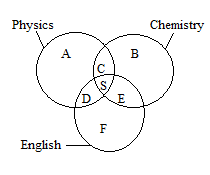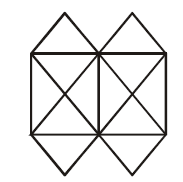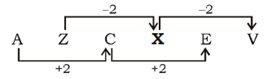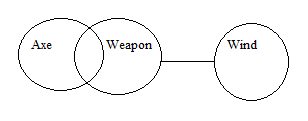# SSC CGL 2018 Practice Test Papers | Reasoning Ability (Day-34)

Dear Aspirants, Here we have given the Important SSC CGL Exam 2018 Practice Test Papers. Candidates those who are preparing for SSC CGL 2018 can practice these questions to get more confidence to Crack SSC CGL 2018 Examination.

[WpProQuiz 3220]

Click “Start Quiz” to attend these Questions and view Explanation

1) Select the related word/letters/number from the given alternatives.

Square: Octagon:: ??

a) Cone : Sphere

b) Triangle : Hexagon

c) Pentagon : Heptagon

2) A Z C ? E V

a) Y

b) D

c) X

d) B

3) The diagram below represents the students who study Physics, Chemistry, and Mathematics.What is the number of students who study both Physics and English?

a) D

b) F

c) A+ D

d) B+C

4) The diagram below represents the students who study Physics, Chemistry, and Mathematics.What is the number of students who study all the three Subjects if  S+ C=10 and S-C=2?

a) 6

b) 4

c) 2

d) 10

5) The diagram below represents the students who study Physics, Chemistry, and Mathematics.What is the sum of Students who study Chemistry alone and English alone?

a) B+C

b) E+D

c) B+F

d) E

6) Correct the following equations by interchanging any two signs.

3 – 9 × 27 + 9 ÷ 3 = 3

a) + and –

b) × and +

c) × and –

d) – and ÷

7) Arrange the following words as per order in the dictionary

i) Atlantic

ii) Acoustic

iii) Amoeba

iv) Arena

a) ii, iii, i, iv

b) ii, iii, iv, i

c) i, ii, iv, iii

d) None of the above

8) From the given alternatives select the word which cannot be formed using the letters of the given word.

CIRCUMSTANCE

a) STAND

b) MAT

c) RUST

d) EAT

9) How many triangles are there in the following figure?a) 20

b) 22

c) 16

d) 24

10) Statement:

Some axe are weapon.

No weapons are wind

Conclusion:

1. Some wind are weapon
2. Some axe are wind

a) Only I follows

b) Only II follows

c) Either I or II follows

d) Neither I nor II follows

The  number of sides in a Octagon is double that of a Square. Similarly The  number of sides in a hexagon is double that of a triangle.number of students who study all the three subjects = S

S+C=10——(i)

S-C=2——–(ii)

On solving (i) and (ii)

S= 6

3 × 9 – 27 + 9 ÷ 3 = 3

27 – 27 + 3 = 3

30 – 27 = 3

3 = 3

ii) Acoustic

iii) Amoeba

iv) Arena

i) Atlantic

D is not there in the given word So STAND cannot be formed.

16 triangles